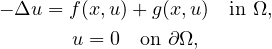Electron. J. Differential Equations, Vol. 2021 (2021), No. 48, pp. 1-12.

### Multiple solutions to boundary value problems for semilinear elliptic equations Duong Trong Luyen, Nguyen Minh Tri

Abstract:
In this article, we study the multiplicity of weak solutions to the boundary value problemwhere Ω is a bounded domain with smooth boundary in RN (N > 2), f(x,ξ) is odd in ξ and g is a perturbation term. Under some growth conditions on f and g, we show that there are infinitely many solutions. Here we do not require that f be continuous or satisfy the Ambrosetti-Rabinowitz (AR) condition. The conditions assumed here are not implied by the ones in [3,15]. We use the perturbation method by Rabinowitz combined with estimating the asymptotic behavior of eigenvalues for Schrödinger's equations.

Submitted September 18, 2019. Published May 28, 2021.
Math Subject Classifications: 35J60, 35B33, 35J25, 35J70.
Key Words: Semilinear elliptic equations; multiple solutions; critical points; perturbation methods; boundary value problem.

Show me the PDF file (341 KB), TEX file for this article.Duong Trong Luyen Division of Computational Mathematics and Engineering Institute for Computational Science, and Faculty of Mathematics and Statistics Ton Duc Thang University, Ho Chi Minh City, Vietnam email: duongtrongluyen@tdtu.edu.vn Nguyen Minh Tri Institute of Mathematics Vietnam Academy of Science and Technology 18 Hoang Quoc Viet 10307 Cau Giay, Hanoi, Vietnam email: triminh@math.ac.vn